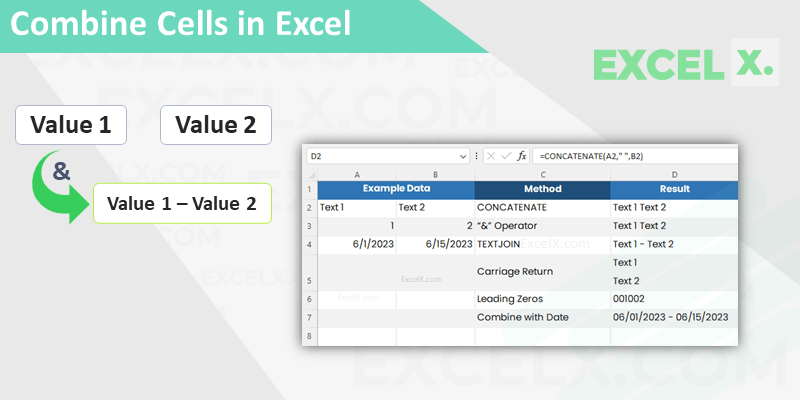# Excel Blog by PNRao

## Convert Numbers to Words in Excel

Converting numbers to words in Excel is a common task, but Excel doesn't have the built-in Function for this. However, it can be easily accomplished

## Excel Formula to Remove Characters From Left

Learn how to remove characters from the left side of a string using Excel formulas such as RIGHT, LEN, and FIND. Improve your data cleaning and enhance productivity with these handy Excel tips.

## Split Text in a Cell – Explained with Examples

Splitting text in Excel is one of the most common tasks in data manipulation process. Microsoft Excel is renowned for its data analysis capabilities

•Combine Cells in Excel
Gallery

## Combine Cells in Excel

Combine Cells in Excel is one of the most used data manipulation tasks. We can combine Cells in Excel in many ways and create combined

## Basic Mathematical Operations in Excel

Excel is a powerful tool for performing basic mathematical operations such as addition, subtraction, multiplication, and division. These operations can be performed using formulas

## Addition in Excel – Explained with Examples

Addition in Excel is a basic arithmetic operation that allows you to add numbers together. Excel provides several ways to add numbers, including using

## Calculating Percentages in Excel

Excel is a powerful tool for performing a variety of calculations, including calculating percentages. Whether you're working with sales data, financial statements, or any

## Excel Project Plan Template

Easily plan your project using Excel project plan template. You can download our free Project Planning Template in Excel Format and create tasks, timelines,

•2023 Calendar in Excel
Gallery

## 2023 Calendar in Excel

2023 Calendar in Excel helps you to create calendar based spreadsheets in Excel. You can download our free Excel Calendar Template 2023, and use